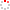#CodeStepByStep## findDominatingSet

Language/Type: C++ Graph collections

Write a function named `findDominatingSet` that looks for a dominating set of a given size in a given graph. For a given graph G, a dominating set is a set of vertexes D where every vertex in G either is an element of D or is adjacent (a neighbor) to an element of D. Another way of phrasing this is that a given set of vertexes D is a dominating set of G if there does not exist any vertex in G outside of D that cannot reach a vertex in D by traveling across a single edge.

Your function accepts three parameters: a reference to a `BasicGraph`, an integer K, and a reference to a `Set` of strings for storing your dominating set (an "output parameter"). Your function should check whether the given graph has any dominating sets that contain no more than K vertexes. If the graph has any such dominating sets, your function should store one of the dominating sets in your output parameter and should return `true`. You should also print the dominating set as output to the console. If there is no dominating set of the given size, you should return `false`; the contents of the output parameter set do not matter and will be ignored by the caller in such a case. If the graph contains multiple dominating sets of the given size, you may emit any one of them.

You may assume that the graph is undirected, in other words, that the edges are bidirectional between neighboring vertexes. If the given graph and set are both empty, you should return `true`.

For example, given the graph below, the call of `findDominatingSet(graph, 3, set)` would return `true`, and would modify the given set to store a trio of vertexes such as `{"V3", "V6", "V9"}`. The function would also print that set as its single line of console output. For the same graph, the call of `findDominatingSet(graph, 2, set)` would return `false` and would produce no console output, because there is no dominating set with ≤ 2 vertexes.We suggest that you first solve the previous problem, `isDominatingSet`, and use that code to help you solve this problem.

Constraints: You may define private helper functions if so desired, and you may construct auxiliary collections as needed to solve this problem. You should not modify the structure of the graph (e.g., add or remove vertexes or edges), but you may wish to modify the state variables inside individual vertexes/edges (e.g., cost, visited, color, etc.).

Type your C++ solution code here:

This is a function exercise. Write a C++ function as described. Do not write a complete program; just the function(s) above.